Courses

Basic CT & DT Signals Notes | Study Digital Signal Processing - Electrical Engineering (EE)

Electrical Engineering (EE): Basic CT & DT Signals Notes | Study Digital Signal Processing - Electrical Engineering (EE)

The document Basic CT & DT Signals Notes | Study Digital Signal Processing - Electrical Engineering (EE) is a part of the Electrical Engineering (EE) Course Digital Signal Processing.
All you need of Electrical Engineering (EE) at this link: Electrical Engineering (EE)

To test a system, generally, standard or basic signals are used. These signals are the basic building blocks for many complex signals. Hence, they play a very important role in the study of signals and systems.

Unit Impulse or Delta Function

A signal, which satisfies the condition, δ(t)=limϵ→∞x(t) is known as unit impulse signal. This signal tends to infinity when t = 0 and tends to zero when t ≠ 0 such that the area under its curve is always equals to one. The delta function has zero amplitude everywhere excunit_impulse.jpgept at t = 0.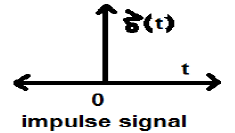Properties of Unit Impulse Signal

• δ(t) is an even signal.
• δ(t) is an example of neither energy nor power (NENP) signal.
• Area of unit impulse signal can be written as;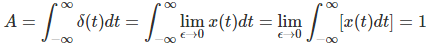• Weight or strength of the signal can be written as;

y(t)=Aδ(t)

• Area of the weighted impulse signal can be written as −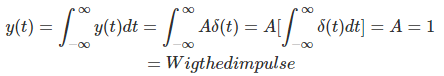Unit Step Signal

A signal, which satisfies the following two conditions −

• U(t)=1(whent≥0)and
• U(t)=0(whent<0)

is known as a unit step signal.

It has the property of showing discontinuity at t = 0. At the point of discontinuity, the signal value is given by the average of signal value. This signal has been taken just before and after the point of discontinuity (according to Gibb’s Phenomena).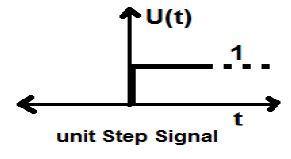If we add a step signal to another step signal that is time scaled, then the result will be unity. It is a power type signal and the value of power is 0.5. The RMS (Root mean square) value is 0.707 and its average value is also 0.5

Ramp Signal

Integration of step signal results in a Ramp signal. It is represented by r(t). Ramp signal also satisfies the condition r(t) = ∫t−∞U(t)dt = tU(t)r(t). It is neither energy nor power (NENP) type signal.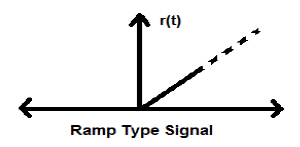Parabolic Signal

Integration of Ramp signal leads to parabolic signal. It is represented by p(t). Parabolic signal also satisfies he condition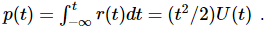It is neither energy nor Power (NENP) type signal.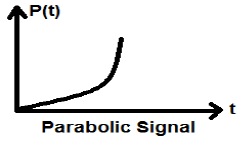Signum Function

This function is represented as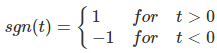It is a power type signal. Its power value and RMS (Root mean square) values, both are 1. Average value of signum function is zero.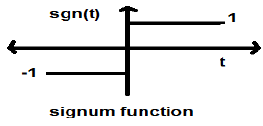Sinc Function

It is also a function of sine and is written as −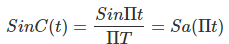Properties of Sinc function

• It is an energy type signal.
•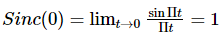•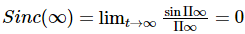(Range of sinπ∞ varies between -1 to +1 but anything divided by infinity is equal to zero)
•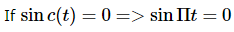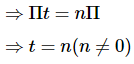Sinusoidal Signal

A signal, which is continuous in nature is known as continuous signal. General format of a sinusoidal signal is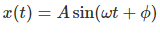Here,

A = amplitude of the signal

ω = Angular frequency of the signal (Measured in radians)

φ = Phase angle of the signal (Measured in radians)

The tendency of this signal is to repeat itself after certain period of time, thus is called periodic signal. The time period of signal is given as;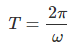The diagrammatic view of sinusoidal signal is shown below.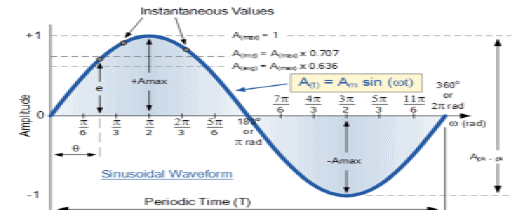Rectangular Function

A signal is said to be rectangular function type if it satisfies the following condition −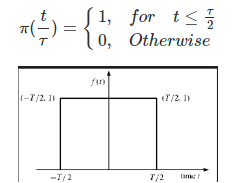Being symmetrical about Y-axis, this signal is termed as even signal.

Triangular Pulse Signal

Any signal, which satisfies the following condition, is known as triangular signal.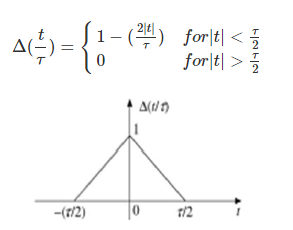This signal is symmetrical about Y-axis. Hence, it is also termed as even signal.

We have seen that how the basic signals can be represented in Continuous time domain. Let us see how the basic signals can be represented in Discrete Time Domain.

Unit Impulse Sequence

It is denoted as δ(n) in discrete time domain and can be defined as;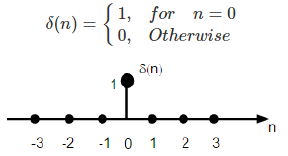Unit Step Signal

Discrete time unit step signal is defined as;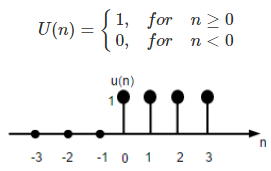The figure above shows the graphical representation of a discrete step function.

Unit Ramp Function

A discrete unit ramp function can be defined as −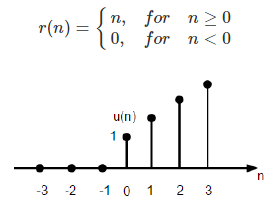The figure given above shows the graphical representation of a discrete ramp signal.

Parabolic Function

Discrete unit parabolic function is denoted as p(n) and can be defined as;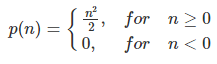In terms of unit step function it can be written as;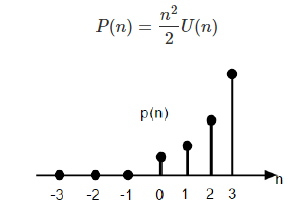The figure given above shows the graphical representation of a parabolic sequence.

Sinusoidal Signal

All continuous-time signals are periodic. The discrete-time sinusoidal sequences may or may not be periodic. They depend on the value of ω. For a discrete time signal to be periodic, the angular frequency ω must be a rational multiple of 2π.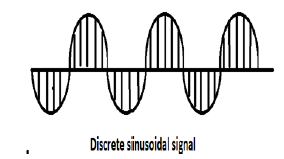A discrete sinusoidal signal is shown in the figure above.

Discrete form of a sinusoidal signal can be represented in the format −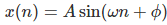Here A,ω and φ have their usual meaning and n is the integer. Time period of the discrete sinusoidal signal is given by −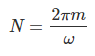Where, N and m are integers.

The document Basic CT & DT Signals Notes | Study Digital Signal Processing - Electrical Engineering (EE) is a part of the Electrical Engineering (EE) Course Digital Signal Processing.
All you need of Electrical Engineering (EE) at this link: Electrical Engineering (EE)Use Code STAYHOME200 and get INR 200 additional OFF Use Coupon Code

Top Courses for Electrical Engineering (EE)Digital Signal Processing

3 videos|50 docs|54 tests

Top Courses for Electrical Engineering (EE)Track your progress, build streaks, highlight & save important lessons and more!

,

,

,

,

,

,

,

,

,

,

,

,

,

,

,

,

,

,

,

,

,

;